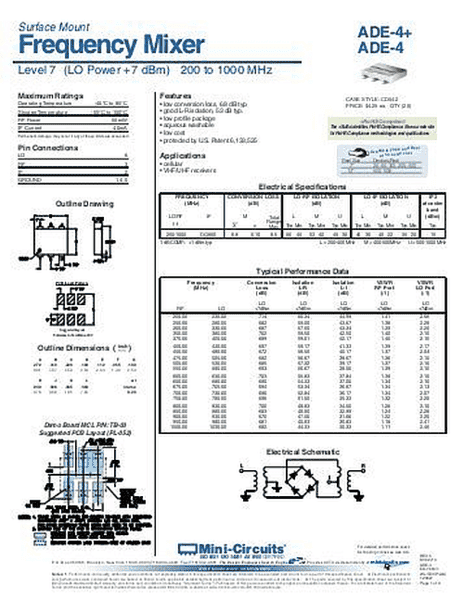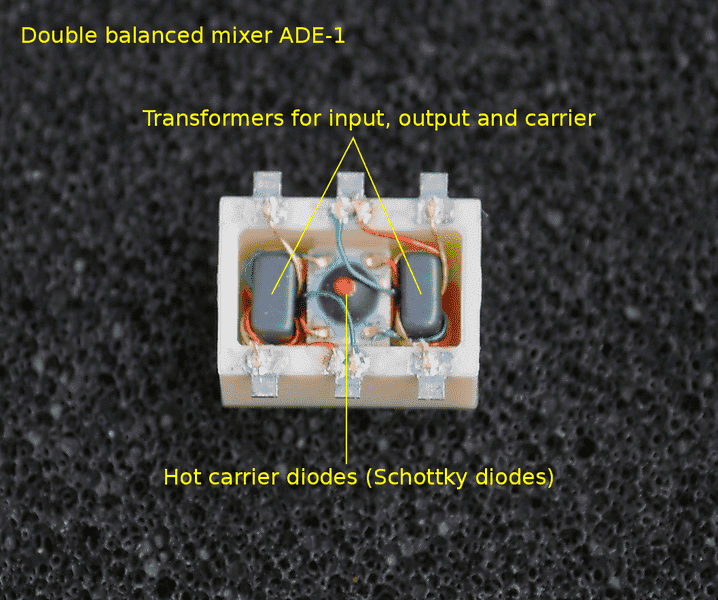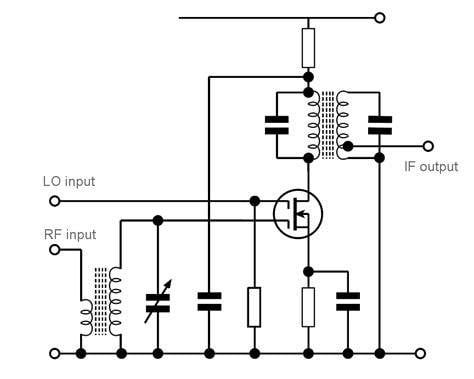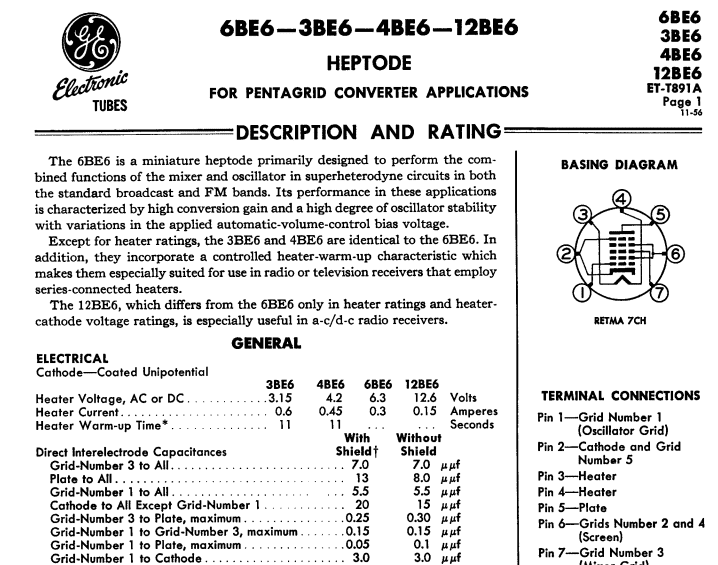# Basic Radio Questions -- Oscillators, Mixers and Modulators

revv
1 - Is a carrier wave always made by some kind of oscillating/oscillator circuit?

2 - Does modulation "modify" or better "modulates" the carrier wave to change the audio signal to amplitude or frequency modulated?

3 - What is the difference between a "radio mixer" and "modulator" do they have anything in common or not?

•berkeman
revv
1 - I believe that an oscillator circuit creates a "pure" sinusoidal wave that is constant in frequency.

2 - I think modulation is a process that "modifies or modulates" a signal containing information from the carrier wave to be modulated by amplitude or frequency.

3 - This I am not so sure about I tried reading the wikipedia and other sources but still don't understand.

•davenn and Greg Bernhardt
Staff Emeritus
Good research. Did you see this?
https://en.wikipedia.org/wiki/Frequency_mixer said:
Frequency mixers are also used to modulate a carrier signal in radio transmitters.

Amplitude modulation methods are very different than frequency modulation differences.

But in both AM and FM radios mixers are used for:
https://en.wikipedia.org/wiki/Frequency_mixer said:
Mixers are widely used to shift signals from one frequency range to another, a process known as heterodyning, for convenience in transmission or further signal processing. For example, a key component of a superheterodyne receiver is a mixer used to move received signals to a common intermediate frequency.

Other PF members are much better radio experts than I am. Wait a bit and you'll get more good answers.

Gold Member
Mathematically, amplitude modulation and frequency mixing are the same. The signals are multiplied together.

Gold Member
Mathematically, amplitude modulation and frequency mixing are the same. The signals are multiplied together.
Although multiplication is involved in both, I think we should expand on that statement. The formula for amplitude modulation is more complicated than just a multiplication because the resulting DSB AM signal has the 'right' amount of carrier to produce a proper envelope. Simple multiplication will just give a 'row of beads' waveform. This link has images of waveforms from both processes. Using the simplest representation, with A being the 'carrier' and B being the modulating waveform
V = A.B simple Multiplication
V = A.(C+B) Amplitude modulation involves adding a constant value C to avoid the envelope dipping below the t axis.
For an RF mixer, a carrier is not needed so a simple multiplication circuit is better and one of the sidebands is chosen (selectively filtered)
There's another sort of fairly obvious difference in that the mixing process can involve a big difference in frequencies (hundreds of MHz received signal and perhaps 10MHz IF frequency) whereas the AM spectrum only extends by (twice) the baseband bandwidth.

•anorlunda
Gold Member
It may also be worth pointing out that you can't get true 'Multipliers' to operate at the higher RF frequencies. Instead, a more simple non-linear device like a diode is often used. This will produce a whole spectrum of non linear products and the wanted product is then selected with a band pass filter.

•davenn, Tom.G and Averagesupernova
Gold Member
Mathematically, amplitude modulation and frequency mixing are the same. The signals are multiplied together.

that is rarely true for mixing
Frequency mixing is more commonly additive or subtractive eg...

I have an incoming freq of 50MHz and I want to down mix to 10.7 Intermediate frequency
I use a local oscillator of 39.3MHz and mix that with the 50MHz.
I get two main products
50 + 39.3 = 89.3
and
50 - 39.3 = 10.7
I use a 10.7 MHz filter to get a clean 10.7MHz output from the mixer
That is an example of the most common use of a mixer in zillions of comms systems
There is no multiplication there, just addition and subtraction

It may also be worth pointing out that you can't get true 'Multipliers' to operate at the higher RF frequencies. Instead, a more simple non-linear device like a diode is often used. This will produce a whole spectrum of non linear products and the wanted product is then selected with a band pass filter.

That is true, us amateurs loved the good old BAY96 power varactor diode for tripling 144MHz to 432MHz or 432MHz to 1296MHz

It would be probably difficult to find a true multiplier ... all the ones I have ever worked with are all just harmonically derived.
Either by diode as above or by interstage transformers where the secondary is tuned for the required harmonic generated by the previous stage

a common line up in a 144MHz amateur transmitter

12MHz x 3 = 36 x 2 = 72 x 2 = 144MHz

there is just inferred multiplication ... it's just the wanted harmonic being picked off at each stage
up the transmitter chain

Dave

Gold Member
Frequency mixing is more commonly additive or subtractive eg...
Wouldn't that imply that an RF mixer could be done with two resistors? As in a passive audio mixer?

I seem to recall that frequency conversion requires a non-linearity.

Gold Member
Wouldn't that imply that an RF mixer could be done with two resistors?

don't think so, well not that I have ever seen
all the mixers I have ever used are passive as in there is no additional power supply added
The mixers are usually made up of a diode bridge and inductors ... eg the much used Mini-circuits brand

eg... just one exampleDave

#### Attachments

Gold Member
Ohhhh almost forgot
one other common form of mixer I have seen/used/worked on is using a dual gate MOSFET
RF in on one gate, LO in on the other gate and the output out of the drain

a representative circuit of that system.#### Attachments

Gold Member
but the mixers are usually made up of a diode bridge and inductors
Yeah, the diodes supply the non-linearity as @sophiecentaur mentioned above.

Just as a curiousity, here is a 4 quadrant analog multiplier good to 250MHz:

You snuck a couple posts in while I was typing!

The double-gate FET mixer, like the old-time vacuum tube mixer, effectively does an analog multiply of the two input signals. If you think about it, one grid/gate will limit the current, while the other one has only that limited current to work on.

Gosh, another entry! I better stop typing and post.

•davenn
Gold Member
Frequency mixing which creates sum and difference frequencies is a result of MULTIPLICATION. Make a couple sine tables in a spreadsheet, multiply them together and see what you get. The sine waves themselves are multiplied together.
-
@sophiecentaur has a very good point in that it is not practical to actually multiply several sine waves together in the 100s of MHz regions so it is done with diode mixers, etc. Also, there are times we don't want 100% modulation so this is a difference between a practical modulator and a practical frequency mixer. However the result of the addition of sum and difference (new) frequencies is the same in AM and a frequency mixer such as what we would use to convert a signal down to an IF.
-
Active mixers are not uncommon at all. Mini circuits double balanced mixers are widely known but there are plenty of examples of single transistor mixers. And don't forget about the good old 1496.

Last edited:
•sophiecentaur
Gold Member
Frequency mixing which creates sum and difference frequencies is a result of MULTIPLICATION. Make a couple sine tables in a spreadsheet, multiply them together and see what you get. The sine waves themselves are multiplied together.

so where is the multiplication in ...

I have an incoming freq of 50MHz and I want to down mix to 10.7 Intermediate frequency
I use a local oscillator of 39.3MHz and mix that with the 50MHz.
I get two main products
50 + 39.3 = 89.3
and
50 - 39.3 = 10.7
I use a 10.7 MHz filter to

50 x 39.3 = 1965 ??

Gold Member
so where is the multiplication in ...

50 x 39.3 = 1965 ??
Don't multiply the frequency. Multiply the sine waves themselves. You didn't put a couple of sine tables into a spreadsheet and graph it did you. I don't know of a more convincing method of proving my point. Why do you think they are called products?

Gold Member
Don't multiply the frequency. Multiply the sine waves themselves. You didn't put a couple of sine tables into a spreadsheet and graph it did you. I don't know of a more convincing method of proving my point.

Or have a computer do it and do an FFT on the result.

•Averagesupernova
Gold Member
Mixing sine waves shows that signal multiplication results in frequency translation. Let ##A = e^{i\omega_1 t}## and ##B= e^{i\omega_2 t}## then

##A * B = e^{i(\omega_1+\omega_2)t}##​

which is a wave at the sum frequency. Since real signals ##\cos( \omega t) = \frac{1}{2}(e^{i\omega t} + e^{-i\omega t})## both sum and differences of frequencies occur. With real devices more terms appear from nonlinearities.

•sophiecentaur and dlgoff
Gold Member
that is rarely true for mixing
Frequency mixing is more commonly additive or subtractive eg...
It depends on what you mean by "adding and subtracting". The mixing products are a result of the product of two signals and the trig identity
SinA . SinB = 1/2(Cos(A-B) -Cos(A+B)) ; the well known sum and difference frequencies
A non linearity can often be expressed as a polynomial
V = ΣPnm V1V2 (apologies to Mathematicians for the sloppy terminology but the meaning is there)
where Pnmis the weighting factor and V1 V2 are the two input signals and that gives you a whole family of Sin(nω1t)Sin(mω2t) components - giving Cos(ω12) and the rest of the components.

•Averagesupernova
Gold Member
don't think so, well not that I have ever seen
all the mixers I have ever used are passive as in there is no additional power supply added

It is addition in "frequency space" (Fourier space) but this is -as has been pointed out above- the result of multiplication in time.

The reason for why you can use a diode as a mixer is that its characteristics (near some working point) means that it will approximately square the voltage (which formally is a Taylor expansion of the "true" exponential I-V characteristics of a diode)., if this is the sum of the two signals you will get I=V^2=(V1+V2)^2
IfV1+V2 is the sum of two sine wave (one being the carrier) this will result in multiplication; which in frequency space results in three components: the carrier, the sum and the difference (plus additional harmonics). By adding more diodes and components you can get more complicated results; eg. IQ mixers configured as single sideband modulators etc,.

All non-linear components can act as mixers; and the more non-linear the better (usually). This is why things like superconducting mixers are used in radio astronomy, where the non-linearity comes from Josephson junctions (which are extremely non-linear).

It is also the reason for why it is sometimes a good idea to dc-bias a mixer; this will shift the working point which can make it behave more or less ideal.

•Integral and Averagesupernova
Gold Member
And then there are Analogue Four Quadrant Multiplier circuits which literally take two voltages and produce the product without any unwanted spectral 'extras'. The principle is that the signal into one circuit controls the current into another circuit in which the input resistance is controlled by the other input signal. Then the resulting Voltage is IR (a product of two signals). It's more complicated than that because the circuit has to work about a 'Zero', to take care of both possible signs of both input signals but the basic V=IR does the true linear multiplication function. Dead clever but it only works at low frequencies. Great for Dalek Voices -"Exterrrrminate!" and modern music effects.

•jim hardy and Paul Colby
Gold Member
Dearly Missed
And then there are Analogue Four Quadrant Multiplier circuits

They're amazingly precise at DC, but i never used one in radio. That one could handle the AM broadcast band...

I'm still living in the days of 6BE6 pentagrid converter. .. works as Sophie described .
http://rtellason.com/tubedata/6BE6.pdf#### Attachments

•dlgoff, sophiecentaur and Paul Colby
Homework Helper
Gold Member
Yeah, the diodes supply the non-linearity as @sophiecentaur mentioned above.
Additionally, it's not the diodes' nonlinear behavior that produces mixing in a double-balanced diode mixer, e.g. a typical Mini-Circuits unit. In fact ideal diodes would work better than real ones.

The diode bridge acts as a chopper, chopping up the input by the reference which is ideally a square wave. So we're multiplying an input sine wave (typically) by a square wave. Fourier series analysis yields all the harmonics which are copious!

Homework Helper
Gold Member

They're amazingly precise at DC, but i never used one in radio. That one could handle the AM broadcast band...

I'm still living in the days of 6BE6 pentagrid converter. .. works as Sophie described .
http://rtellason.com/tubedata/6BE6.pdf
View attachment 224317
The spec sheet is a poignant reminder of the glory days of GE before they became a bloated conglomerate including finance and entertainment. I still have two editions of the GE Transistor Manual - irreplaceable!

Gold Member
In fact ideal diodes would work better than real ones.
An ideal diode characteristic is still non-linear. A linear characteristic would be a diagonal line through the origin of a VI curve (as for a resistor. 'Curved' is just one version of non-linearity.

Homework Helper
Gold Member
An ideal diode characteristic is still non-linear. A linear characteristic would be a diagonal line through the origin of a VI curve (as for a resistor. 'Curved' is just one version of non-linearity.
Yes. I just wanted to dispel the notion that it's the nonlinear characteristic in the forward region that's relevant.

•sophiecentaur
dougfgd
Non-linearity is at the root of all mixers and becomes distortion if it is unwanted .
the parameters that are non linear can be resistance, or capacitance. Oscillators are created by negative resistance which is like negative friction, somewhere there is a wonderful simulation where friction is removed from chair to floor interface, So the chair spontaneously moves from wall to wall.
Fast Radio Bursts must have started as an oscillation pumped by a noise source possibly a collapsing supernova. The initial microsecond burst after traveling millions of light years gets stretched to milliseconds long by propagation through slightly ionised interstellar space the stretch is accompanied by chirp modulation which may enable it to be re-compressed to restore signal to noise ratio .